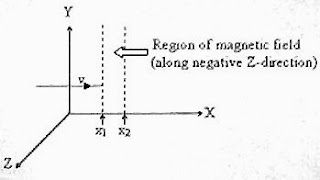## Pages

`“Life is like riding a bicycle.  To keep your balance you must keep moving.”–Albert Einstein`

## Wednesday, February 13, 2008

### AP Physics B & C- Answer to Free-Response Question on Magnetic Fields

"Only two things are infinite, the universe and human stupidity, and I'm not sure about the former."
- Albert Einstein

A free response question (for AP Physics B & C aspirants) involving magnetic fields was posted on 11th February 2008 for your practice. This was the question:

(a) An electron enters an environment of a non-uniform static magnetic field varying in magnitude and direction from point to point, and comes out of it following an irregular path. Would its final speed be equal to the initial speed if it suffered no collisions with anything during its irregular motion in the magnetic field? Justify your answer.
(b) In a cloud chamber photograph of the electron-positron pair production by a high energy gamma ray photon, two circular tracks emerging from a common point can be seen. The tracks curve in opposite directions in a plane normal to the magnetic field applied in the chamber. But if this event is photographed in a liquid hydrogen bubble chamber, two spiral tracks (instead of circular tracks) are seen. Explain why.
(c) Explain why a charged particle projected into a uniform magnetic field at an arbitrary angle θ with respect to the field follows in general, a helical path. Derive an expression for the ‘pitch’ of the helix.(d) A positively charged particle of mass ‘m’ and charge ‘q’ traveling with constant velocity ‘v’ in the positive X-direction enters a uniform magnetic field B directed along the negative Z-direction extending from x = x1 to x = x2 (fig.). Derive an expression (in terms of the given parameters) for the minimum velocity required for the particle to cross the magnetic field.
As promised, I give the answer below:
(a) The magnetic force on a moving charge is always perpendicular to the magnetic field and hence no work is done by the field. The kinetic energy and the speed of the charged particle therefore remain unchanged.
[You might have noted the adjective ‘static’ with the magnetic field. If the magnetic field has a time variation, there will be induced electromotive force, which will change the speed of the charged particle (as in a betatron)].
(b) In a liquid, the charged particles lose energy by collision to a much greater extent than in a gas. (They cause much greater ionization in a liquid medium). The velocity of the charged particle goes on decreasing and therefore, the radius (r) of the circular path goes on decreasing in accordance with the expression for the radius,
r = mv/qB where ‘m’ is the mass, ‘q’ is the charge and ‘v’ is the speed of the particle and B is the magnetic flux density.Instead of a circular path, a spiral path is therefore followed by the particle.
(c) When a charged particle is projected with velocity ‘v’ in to a magnetic field at some arbitrary angle θ with respect to the field, the velocity component v cosθ, which is along the direction of the magnetic field makes the particle move forward where as the velocity component v sinθ, which is perpendicular to the direction of the magnetic field makes the particle move along a circle of radius ‘r’ given by the equation,
(mv2 sin2 θ)/r = q(v sinθ)×B
[We have equated the centripetal force to the magnetic force].
Therefore, r = (mv sinθ)/qB
The charged particle therefore moves along a helical path within the magnetic field.
The ‘pitch’ of the helix is obtained by multiplying the forward velocity v cosθ with the period (T) of the circular component of motion. The period of the circular component of motion is given by
T = (2πr/v sinθ) = [2π(mv sinθ/qB)] /v sinθ) = 2πm/qB
(d) The charged particle will move along a semicircular path just before crossing the magnetic field (fig.). The direction of the curve is obtained by applying Fleming’s left hand rule for the magnetic force. If the velocity of the particle is slightly greater than the velocity for traversing the semicircle, it will cross the magnetic field. Therefore, the required limiting velocity is the velocity for the motion along the semicircle within the magnetic field region.
The radius of the semicircle is x2 – x1. Therefore, the limiting velocity ‘v’ is given by
mv2/r = qvB where r = x2 – x1
Therefore, mv2/(x2 – x1) = qvB, from which v = qB(x2 – x1)/m